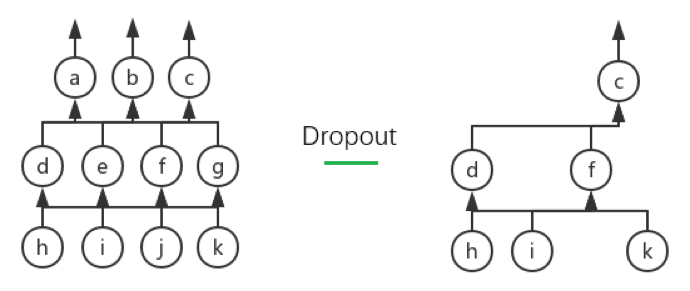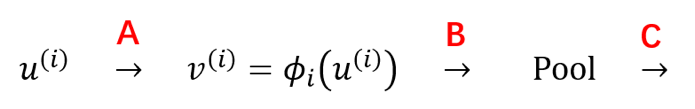# 重写 Layer 结构

• 对于激活函数，只用定义其原始形式、不必定义其导函数形式
• 解决上一章遗留下来的、特殊层结构的实现问题
• 要考虑当前层为 FC（全连接层）时的表现
• 让用户可以选择是否给 Layer 加偏置量

# 实现特殊层

Dropout 的详细说明自然是看原 paper 最好，这里我就大概翻译、总结一下主要内容。Dropout 的核心思想在于提高模型的泛化能力：它会在每次迭代中依概率去掉对应 Layer 的某些神经元，从而每次迭代中训练的都是一个小的神经网络。这个过程可以通过下图进行说明：Dropout 过程的合理性需要概率论上一些理论的支撑，不过鉴于 Tensorflow 框架有封装好的相应函数、我们就不深入介绍其具体的数学原理而仅仅说明其直观（以drop_prob为 50%为例，其余drop_prob的情况是同理的）：

• 在训练过程中，由于 Dropout 后留下来的神经元可以理解为“在 50%死亡概率下幸存”的神经元，所以给将它们对应的输出进行“增幅”是合理的。具体而言，假设一个神经元$n_{i}$的输出本来是$o_{i}$，那么如果 Dropout 后它被留下来了的话、其输出就应该变成$o_{i} \times \frac{1}{50\%} = 2o_{i}$（换句话说、应该让带 Dropout 的期望输出和原输出一致：对于任一个神经元$n_{i}$，设drop_prob$p$而其原输出为$o_{i}$，那么当带 Dropout 的输出为$o_{i} \times \frac{1}{p}$时、$n_{i}$的期望输出即为$p \times o_{i} \times \frac{1}{p} = o_{i}$
• 由于在训练时我们保证了神经网络的期望输出不变、所以在预测过程中我们还是应该让整个网络一起进行预测而不进行 Dropout（关于这一点，原论文似乎也表示这是一种“经试验证明行之有效”的办法而没有给出具体的、原理层面的说明）

BN 打算解决的正是随着前向传导算法的推进、得到的数据的质量会不断变差的问题，它能通过对中间层数据进行某种规范化处理以达到类似对输入归一化处理的效果。事实上回忆上一章的内容、我们已经提到过 Normalize 的核心思想在于把父层的输出进行“归一化”了，下面我们就简单看看它具体是怎么做到这一点的

1. 输入：某一层$L_{i}$在当前 Batch 上的输出$v^{\left( i \right)}$、增强数值稳定性所用的小值$\epsilon$
2. 过程
1. 计算当前 Batch 的均值、方差：
2. 归一化：
3. 线性变换：
3. 输出：规范化处理后的输出$y^{\left( i \right)}$

BN 的核心即在于$\gamma$$\beta$这两个参数的应用上。关于如何利用反向传播算法来更新这两个参数的数学推导会稍显繁复、我们就不展开叙述了，取而代之、我们会直接利用 Tensorflow 来进行相关的实现

Mean）”和“运行方差（Running Variance）”的变量。具体而言：

1. 输入：某一层$L_{i}$在当前 Batch 上的输出$v^{\left( i \right)}$、增强数值稳定性所用的小值$\epsilon$；动量值$m$（一般取$m = 0.9$
2. 过程
首先要初始化 Running Mean、Running Variance 为 0 向量： 并初始化$\gamma$$\beta$为 1、0 向量： 然后进行如下操作：
1. 计算当前 Batch 的均值、方差：
2. 利用$\mu_{i}$$\sigma_{i}^{2}$和动量值$m$更新$\mu_{run}$$\sigma_{run}^{2}$
3. 利用$\mu_{run}$$\sigma_{run}^{2}$规范化处理输出：
4. 线性变换：
3. 输出：规范化处理后的输出$y^{\left( i \right)}$

• 无论是上述的哪种算法、BN 的训练过程和预测过程的表现都是不同的。具体而言，训练过程和算法中所叙述的一致、均值和方差都是根据当前 Batch 来计算的；但测试过程中的均值和方差不能根据当前 Batch 来计算、而应该根据训练样本集的某些特征来进行计算。对于第二个算法来说，$\mu_{\text{run}}$$\sigma_{\text{run}}^{2}$天然就是很好的、可以用来当测试过程中的均值和方差的变量，对于第一个算法而言就需要额外的计算
• 对于 Normalize 这个特殊层结构来说、偏置量是一个冗余的变量；这是因为规范化操作（去均值）本身会将偏置量的影响抹去、同时 BN 本身的$\beta$参数可以说正是破坏对称性的参数，它能比较好地完成原本偏置量所做的工作
• Normalize 这个层结构是可以加在许多不同地方的（如下图所示的 A、B 和 C 处），原论文将它加在了 A 处、但其实现在很多主流的深层 CNN 结构都将它加在了 C 处；相对而言、加在 B 处的做法则会少一些# 重写网络结构

• 初始化各个权值矩阵时，从初始化为 Numpy 数组改为初始化为 Tensorflow 数组、同时要注意兼容 CNN 的问题
• 不用记录所有 Layer 的激活值而只用关心输出 Layer 的输出值和 CostLayer 的损失值（在上个系列中、我们是要记录所有中间结果以进行反向传播算法的）